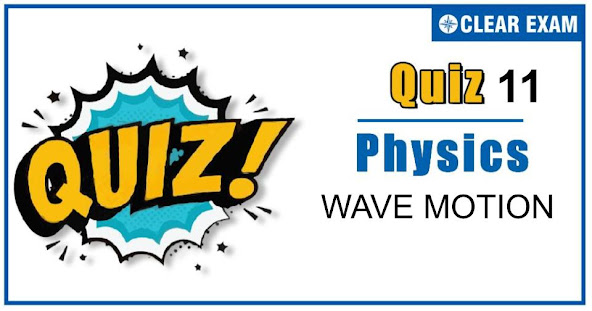## WAVES MOTION QUIZ-11

As per analysis for previous years, it has been observed that students preparing for NEET find Physics out of all the sections to be complex to handle and the majority of them are not able to comprehend the reason behind it. This problem arises especially because these aspirants appearing for the examination are more inclined to have a keen interest in Biology due to their medical background. Furthermore, sections such as Physics are dominantly based on theories, laws, numerical in comparison to a section of Biology which is more of fact-based, life sciences, and includes substantial explanations. By using the table given below, you easily and directly access to the topics and respective links of MCQs. Moreover, to make learning smooth and efficient, all the questions come with their supportive solutions to make utilization of time even more productive. Students will be covered for all their studies as the topics are available from basics to even the most advanced. .

Q1. When a sound wave of wavelength λ is propagating in a medium, the maximum velocity of the particle is equal to the velocity. The amplitude of wave is
•  λ
•  λ/2
•  λ/2π
•  λ/4π
Solution
Given, vmax⁡ =v ⟹ aω=v ⟹a×2πv=vλ or a=λ/2π

Q2. In a medium sound travels 2 km in 3 sec and in air, it travels 3 km in 10 sec. The ratio of the wavelengths of sound in the two media is
•  1∶8
•  1∶18
•  8∶1
•  20∶9
Solution
v∝λ⇒λ12 =v1/v2 =(2/3)/(3/10)=20/9

Q3. Velocity of sound is maximum in
•  Air
•  Water
•  Vacuum
•  Steel
Solution
Velocity of sound in steel is maximum out of the given materials water and air. In vacuum sound cannot travel, it’s speed is zero

Q4. The fundamental frequency of a sonometre wire is n. If its radius is doubled and its tension becomes half, the material of the wire remains same, the new fundamental frequency will be
•  n
•  n/√2
•  n/2
•  n/(2√2)
Solution
n=1/2l √(T/(πr2 ρ))⇒n∝√T/r ⇒n2/n1 =r1/r2 √(T2/T1 )=1/2×√(1/2)=1/(2√2)

Q5. A band playing music at a frequency f is moving towards a wall at a speedv_b. A motorist is following the band with a speedv_m. If v is speed of sound, the expression for the beat frequency heard by the motorist is
•  (v+vm)f)/(v+vb )
•  (v+vm )f/(v-vb )
•  2vb (v+vm)f/(v2-vb2 )
•  2vm (v+vb)f/(v2-vb2 )
Solution
The motorist receives two sound waves, direct one from the band and that reflected from the wall, figure. For direct sound waves, apparent frequency f^'=((v+vm)f)/(v+vb ) For reflected sound waves. Frequency of sound wave reflected from the wall f''=(v×f)/(v-vb ) Frequency of reflected waves as received by the moving motorist, f'=((v+vm ) f'')/v=((v+vm)f)/(v-vb ) ∴ Beat frequency =f''-f' =((v+vm)f)/(v-vb )-((v+vm)f)/(v+vb)=(2vb (v+vm)f)/(v2-vb2 )

Q6. If the velocity of sound in air is 350 m/s. Then the fundamental frequency of an open organ pipe of length 50 cm, will be
•  350 Hz
•  1.75 Hz
• 900 Hz
•  750 Hz
Solution
Fundamental frequency of open pipe n1=v/2l=350/(2×0.5)=350 Hz

Q7. If the pressure amplitude in a sound wave is tripled, then the intensity of sound is increased by a factor of
•  9
•  3
•  6
•  √3
Solution
Intensity ∝(Amplitude)2

Q8. A police car with a siren of frequency 8 kHz is moving with uniform velocity 36 km/h towards a tall building which reflects the sound waves. The speed of sound in air is 320 m/s. the frequency of the siren heard by the car driver is
•  8.5 kHz
•  8.25 kHz
•  7.25 kHz
•  7.5 kHz
Solution
36 km/h=36×5/18 =10m/s
Apart frequency of sound heard by car driver (observer) f'=f((v+vo)/(v-vs )) =8((320+10)/(320-10)) f'=8.5 kHz

Q9.  A source producing sound of frequency 170 Hz is approaching a stationary observer with a velocity 17 ms(-1). The apparent change in the wavelength of sound heard by the observer is (speed of sound in air =340 ms(-1)
•  0.1m
•  0.2m
•  0.4m
•  0.5m
Solution
λ=v/n=340/170=2m,n'=340/(340-17)×170⇒n'=178.9Hz Now λ'=v/n'=340/178.9=1.9 ⇒λ-λ'=2-1.9=0.1

Q10. Two tuning fork of frequency n_1and n_2 produces n beats per second. If n2 and n are known, n1may be given by
•  n2/n+n2
•  n2 n
•  n2±n
• n2/n-n2
Solution
Beat frequency =number of beats/s. n=n2±n1 ∴n1=n2±n## Want to know more

Please fill in the details below:

## Latest NEET Articles\$type=three\$c=3\$author=hide\$comment=hide\$rm=hide\$date=hide\$snippet=hide

Name

ltr
item
BEST NEET COACHING CENTER | BEST IIT JEE COACHING INSTITUTE | BEST NEET & IIT JEE COACHING: WAVES MOTION QUIZ-11
WAVES MOTION QUIZ-11